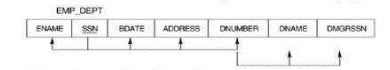# Explain the inference rules for functional dependencies in DBMS

Functional dependencies are the constraints that are derived from the meaning and interrelationship of the data. Let F is a set of all functional dependencies. The set of all dependencies that include F as well as all dependencies that can be inferred from F is called CLOSURE of F denoted by F+.

## Example 1

Given below is an example of functional dependency in database management system (DBMS) −

F= { SSN-> {ENMAE,BDATE,ADDRESS,DNUMBER}, DNUMBER-> {DNAME,DMGRSSN} }

## Output

You will get the following result −## Example 2

Given below is another example of functional dependency in the DBMS −

F+ = { SSN-> {ENAME,BDATE,ADDRESS,DNUMBER},
ENO->ENO,
DNUMBER-> DNAME }

To determine a systematic way to infer dependencies, we must discover a set of interface rules that can be used to infer new dependencies from a given set of dependencies.

## Rules of functional dependencies

There are six inference rules which are as follows −

• Reflexivity: X->X // An attribute(s) determines itself
• Augmentation: if X->Y then XZ->YZ
• Transitivity: if X->Y & Y->Z then X->Z
• Additivity or Union : if X->Y & X->Z then X->YZ
• Projectivity or Decomposition: If X->YZ then X-> Y & X->Z
• Pseudo-Transitivity: If X->Y, YZ->W then XZ->W

## Example

Let’s take a relation R with attributes R(A,B,C,D,E,F)

F: AB->C, BC->AD, D->E, E->F, CF->B, then prove that F logically implies CD->B

## Solutions

D->E, E->F THEN D=>F { Transitivity property}.

D->E, CF-> THEN D=>B {Pseudo-Transitivity}

D->E, E->F THEN D=>F {Transitivity}

D->F THEN CD->CF {Augmentation}

CD->CF, CF-> B THEN => CD-> B {Transitivity}